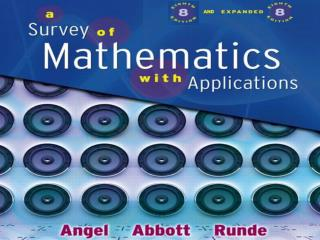# AND - PowerPoint PPT PresentationDownload PresentationAND

ANDDownload Presentation## AND

- - - - - - - - - - - - - - - - - - - - - - - - - - - E N D - - - - - - - - - - - - - - - - - - - - - - - - - - -
##### Presentation Transcript

1. AND

2. Chapter 2 Sets

3. • Methods to indicate sets, equal sets, and equivalent sets • Subsets and proper subsets • Venn diagrams • Set operations such as complement, intersection, union, difference and Cartesian product • Equality of sets • Application of sets • Infinite sets WHAT YOU WILL LEARN

4. Section 1 Set Concepts

5. Set • A collection of objects, which are called elements or members of the set. • Listing the elements of a set inside a pair of braces, { }, is called roster form. • The symbol , read “is an element of,” is used to indicate membership in a set. • The symbol means “is not an element of.”

6. Well-defined Set • A set which has no question about what elements should be included. • Its elements can be clearly determined. • No opinion is associated its the members.

7. Roster Form • This is the form of the set where the elements are all listed, separated by commas. Example: Set A is the set of all natural numbers less than or equal to 25. Solution: A = {1, 2, 3, 4, 5,…, 25} The 25 after the ellipsis indicates that the elements continue up to and including the number 25.

8. Set-Builder (or Set-Generator) Notation • A formal statement that describes the members of a set is written between the braces. • A variable may represent any one of the members of the set. Example: Write set B = {2, 4, 6, 8, 10} in set-builder notation. Solution: The set of all x such that x is a natural number and x is an even number 10.

9. Finite Set • A set that contains no elements or the number of elements in the set is a natural number. Example: Set S = {2, 3, 4, 5, 6, 7} is a finite set because the number of elements in the set is 6, and 6 is a natural number.

10. Infinite Set • An infinite set is a set where the number of elements is not a natural number; that is, you cannot count the number of elements. • The set of natural numbers is an example of an infinite set because it continues to increase forever without stopping, making it impossible to count its members.

11. Equal Sets • Equal sets have the exact same elements in them, regardless of their order. • Symbol: A = B • Example: { 1, 5, 7 } = { 5, 7, 1 }

12. Cardinal Number • The number of elements in set A is its cardinal number. • Symbol: n(A) • Example: A = { 1, 5, 7, 10 } n(A) = 4

13. Equivalent Sets • Equivalent sets have the same number of elements in them. • Symbol: n(A) = n(B) • Example: A = { 1, 5, 7 } , B = { 2, 3, 4 } n(A) = n(B) = 3 So A is equivalent to B.

14. Empty (or Null) Set • The null set (or empty set )contains absolutely NO elements. • Symbol:

15. Universal Set • The universal set contains all of the possible elements which could be discussed in a particular problem. • Symbol: U

16. CourseSmart Page 50